Mechanics

# Conservation of mechanical energy

The conservation of mechanical energy is a principle of physics that ensures that in the absence of dissipative forces such as friction, the total amount of energy in a system never changes . According to the conservation of mechanical energy, the sum of the kinetic energy and the potential energies must have a constant magnitude.

## Conservation of mechanical energy

When a system is completely free from frictional forces or drag forces, the mechanical energy of that system will be constant . This means that a pendulum free from frictional forces, for example, must oscillate indefinitely, otherwise, in a finite time, this pendulum will have its energy dissipated in other forms of energy, such as thermal energy, vibrations, sounds, etc. .

Observe the following figure, in it we have a mobile that moves with constant speed , free of friction forces with the ground, with the air and free of friction forces between its components. In this case, we say that the mechanical energy associated with that body will be equal at points A, B, and C.

At point A, the car has both kinetic and potential energy, thanks to its small height above the lowest level of the ground. Already at point B, the car approaches a situation where all its kinetic energy becomes gravitational potential energy, in other words, as the kinetic energy of the vehicle decreases, its gravitational potential energy increases, as we wrote in following formula, which relates the mechanical energies of points A and B: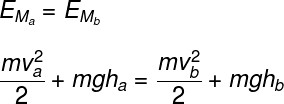a – velocity of the body in position A (m/s)

b – velocity of the body in position B (m/s)

g – gravity (m/s²)

a – height of point A (m)

b – height of point B (m)

As this theme addresses different types of energy, in the following topics, we bring brief definitions of those considered most common in high school, in order to review this content and provide a more complete learning.

## mechanical energy

The mechanical energy of a system is defined as the sum of the kinetic energy with the different potential energies present there, such as gravitational potential energy or elastic potential energy (these being the most common in exercises carried out in high school), among others.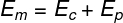M – mechanical energy (J)

C – kinetic energy (J)

P – potential energy (J)

When there is friction, a part of the mechanical energy of the system is “lost”, being converted into a thermal agitation of the atoms and molecules. This type of energy resulting from the action of the friction force is the thermal energy of the body, and its correspondence with heat was explained by James Prescott Joule , through his experiment on the mechanical equivalence of heat.

Kinetic energy

Kinetic energy is the form of energy related to the motion of a body. It is a scale quantity r, proportional to the mass of the body and the square of its velocity, in units of the International System of Units ( SI ), it is measured in joules (J) and can be calculated using the following formula: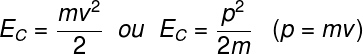p – momentum (kg.m/s)

m – mass (kg)

## Potential energy

Potential energy is the generic name given to any form of energy that can be stored. These energies only arise when conservative forces are applied . Examples of potential energy are:

• Gravitational potential energy : form of energy generated when some body presents a certain height in relation to the Earth’s surface.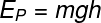• Elastic potential energy : form of energy related to the deformation of elastic bodies, which tend to return to their original shape after being deformed.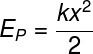k – spring constant (N/m)

x – deformation (m)

• Electric potential energy: is the energy that arises through the attractive or repulsive interaction between electric charges .

## Exercises solved

Question 1) (G1 – IFBA) A body is dropped from the top of an inclined plane, as shown in the figure below. Assuming ideal polished surfaces, zero air resistance, and 10 m/s 2 as the local acceleration due to gravity, determine the approximate speed with which the body strikes the ground:

a) v = 84 m/s

b) v = 45 m/s

c) v = 25 m/s

d) v = 10 m/s

e) v = 5 m/s

Solution:

alternative d.

To determine the approximate speed with which the body reaches the ground, we must apply the principle of conservation of mechanical energy. Therefore, we say that the gravitational potential energy at the top of the inclined plane is equal to the kinetic energy of this body at the bottom of the plane.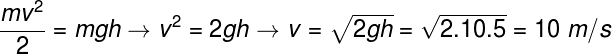Upon resolution, the masses present on both sides of the equation cancel out. Then, we replaced the values ​​informed by the statement and did some algebraic operations until we found the speed of 10 m/s.

Question 2) (UEG) In an experiment that validates the conservation of mechanical energy, a 4.0 kg object collides horizontally with a relaxed spring with a spring constant of 100 N/m. This shock compresses her 1.6 cm. What is the speed, in m/s, of this object before it hits the spring?

a) 0.02

b) 0.40

c) 0.08

d) 0.13

Solution

alternative c.

In this exercise, we say that the kinetic energy of the body is fully converted into elastic potential energy, so we must make the following calculation: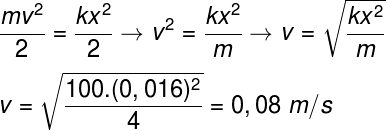Question 3) (G1 – IFSP) A pole vaulter, during his run to overcome the obstacle in front of him, transforms his ____________ energy into ____________ energy due to the height gain and consequently to the _____________ of his speed.

The gaps in the text above are correctly and respectively filled in by:

a) potential – kinetic – increase

b) thermal – potential – decrease

c) kinetic – potential – decrease

d) kinetic – thermal – increase

e) thermal – kinetic – increase

Solution

alternative c.

A pole vaulter, during his race to cross the obstacle in front of him, transforms his kinetic energy into potential energy due to the increase in height and consequently to the decrease in his speed.